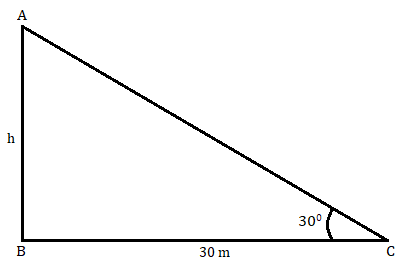# The angle of elevation of the top of tower, from the point on the ground and at a distance of 30 m from its foot, is 30o. Find the height of tower.

Given:  The angle of elevation of the top of tower, from the point on the ground and at a distance of 30 m from its foot, is 30o

To find: We have to find the height of tower.

Solution:In the above image AB represents the tower, C is the point at a distance of 30 m from foot of the tower and the angle of elevation of the top of tower from point C is 30o.

Let height of tower = h metre

Now,

In ABC:

$tan\ 30\ =\ \frac{h}{30}$

$\Longrightarrow \ \frac{1}{\sqrt{3}} \ =\ \frac{h}{30}$

$\Longrightarrow \ h\ =\ \frac{30}{\sqrt{3} \ } \ =\ 10\sqrt{3}$

So, height of tower = h = 103 m

Updated on: 10-Oct-2022

80 Views